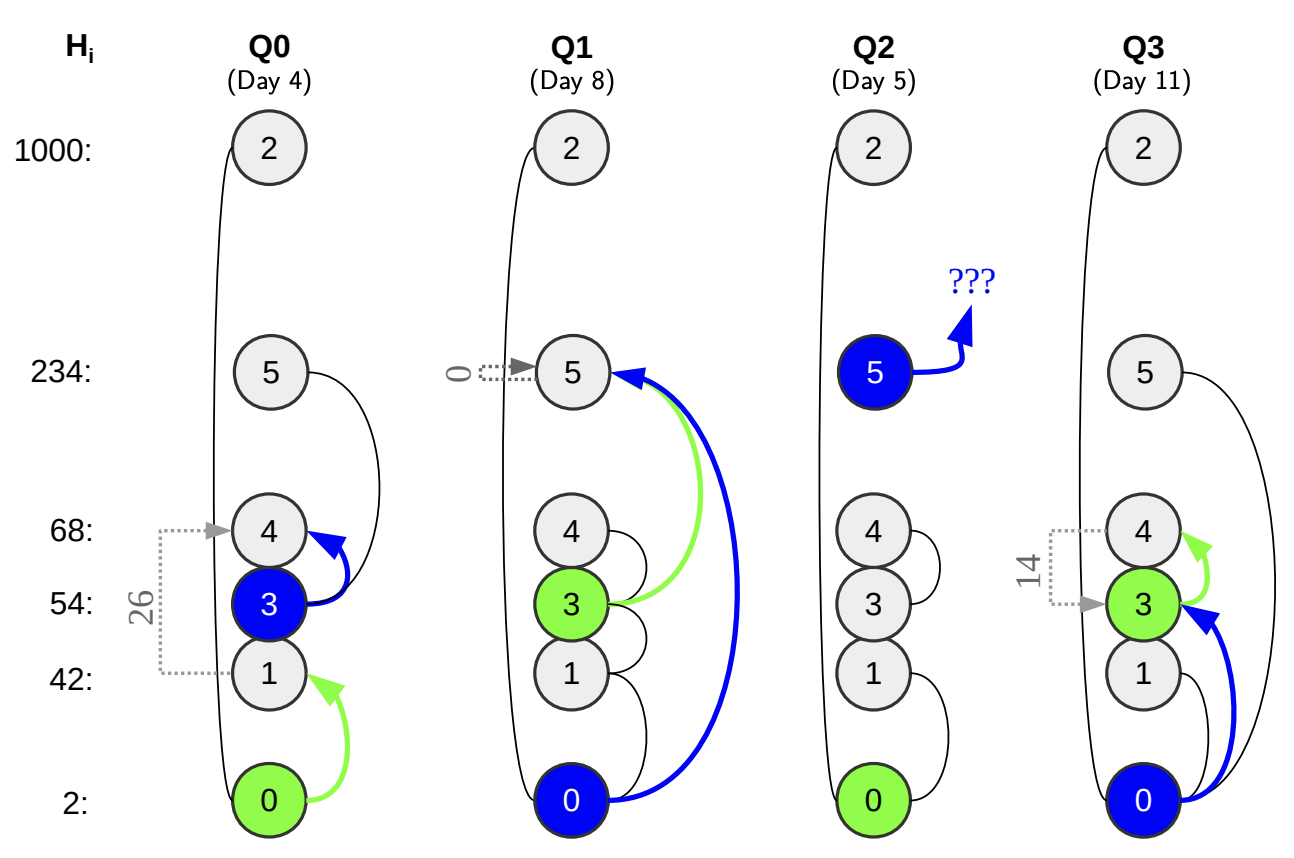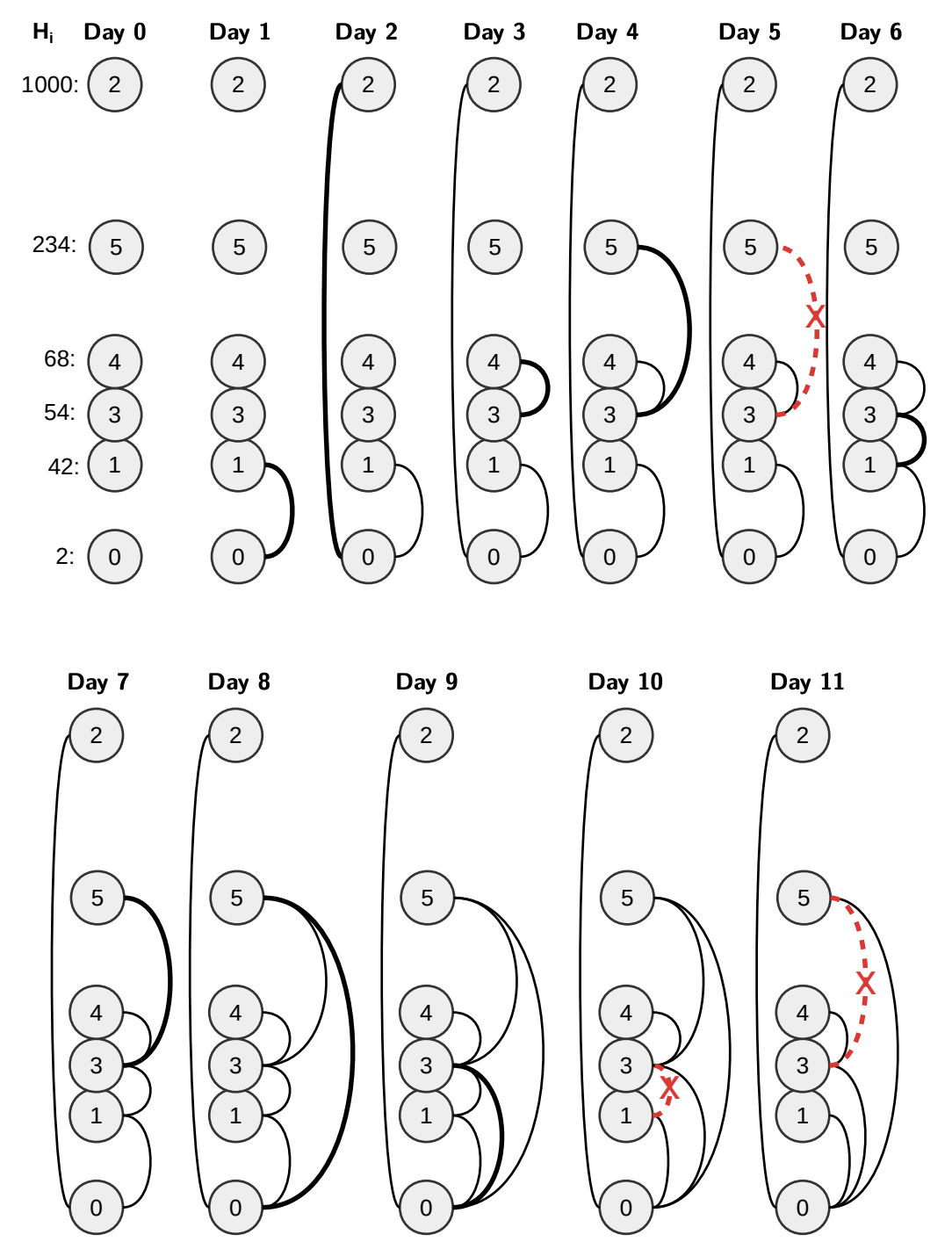시간 제한 메모리 제한 제출 정답 맞은 사람 정답 비율
3 초 256 MB 20 3 3 60.000%

## 문제

Once upon a time, in the Land of the Shamans, everyone lived on the Sky-High Beanstalk. Each shaman had a unique identifying number i between 0 and N − 1, and an altitude value Hi, representing how high he lived above ground level. The distance between two altitudes is the absolute value of their difference.

All shamans lived together in peace, until one of them stole the formula of the world-famous Potion of Great Power. To cover his/her tracks, the Thief has put a Curse on the land: most inhabitants could no longer trust each other. . .

Despite the very difficult circumstances, the Order of Good Investigators have gained the following information about the Curse:

• When the Curse first takes effect everyone stops trusting each other.
• The Curse is unstable: at the end of each day (exactly at midnight), one pair of shamans will start or stop trusting each other.
• Unfortunately, each shaman will only ever trust at most D others at any given time.

They have also reconstructed a log of who trusted whom: for each night they know which pair of shamans started/stopped trusting each other.

They believe the Thief has whispered the formula to an Evil Shaman. To avoid detection, both of them visited the home of one of their (respective) trusted friends. During the visit, the Thief whispered the formula to the Evil Shaman through the window. (Note: this trusting friend did not have to be home at the time. In fact, it’s even possible that they visited each other’s houses – shamans are weird.)

Fortunately, whispers only travel short distances, so the Order knows the two trusting friends visited (by the Thief and the Evil Shaman) must live very close to each other.

They ask you to help with their investigation. They would like to test their suspicions: what if the Thief was x, the Evil Shaman was y, and the formula was whispered on day v? What is the smallest distance the whispered formula had to travel? That is, what is the minimum distance between the apartments of some shamans x' and y' (i.e. min(|Hx'Hy'|)), such that x' was a trusted friend of x and y' was a trusted friend of y on day v?

They will share all their information with you, then ask you a number of questions. You need to answer each question immediately, before receiving the next one.

## 라이브러리

This is an interactive task. You should implement the following functions:

• void init(int N, int D, int H[])
N is the number of shamans, D is the maximum number of trusted friends a shaman can have at any given point, and H is an array of size N, where H[i] denotes the altitude of shaman i (for 0 ≤ i < N).
• void curseChanges(int U, int A[], int B[])
U is the number of days, and A and B are arrays of size U, such that A[i] and B[i] are the pair of shamans who started or stopped trusting each other at the end of day i (for 0 ≤ i < U). That is, if A[i] and B[i] trusted each other on day i, they did not trust each other on day i + 1, or vice versa.
• int question(int X, int Y, int V)
X is the suspected Thief, Y is the suspected Evil Shaman, and V is the suspected day. You should return the minimum distance the whispered formula had to travel from some trusted friend X' of X to a trusted friend Y' of Y
In case someone trusted both X and Y (i.e. X' = Y'), you should return 0. If X or Y had no trusting friends, return 109.

The first two functions will each be invoked exactly once, in the order they appear above, at the beginning of program execution. Following this, the question function will be invoked multiple times. Denote the number of invocations by Q.

## 제한

• 2 ≤ N ≤ 105
• 1 ≤ D ≤ 500
• 0 ≤ U ≤ 2 · 105
• 1 ≤ Q ≤ 50 000
• 0 ≤ Hi ≤ 109 for all i (0 ≤ i < N).
• 0 ≤ A[j], B[j], X, Y < N and XY and A[j] ≠ B[j] for all j (0 ≤ j < U)
• 0 ≤ VU

## 예시

init  N=6, D=5, H={ 2, 42, 1000, 54, 68, 234 }) ;

// Day:                1. 2. 3. 4. 5. 6. 7. 8. 9.10.11.
curseChanges(U=11, A={ 0, 2, 3, 3, 3, 1, 5, 0, 3, 1, 3 },
B={ 1, 0, 4, 5, 5, 3, 3, 5, 0, 3, 5 });

question(X=0, Y=3, V= 4) returns 26;
// Explanation: |H-H| = 26

question(X=3, Y=0, V= 8) returns 0;
// Explanation: |H-H| = 0 or |H-H| = 0

question(X=0, Y=5, V= 5) returns 1000000000;
// Explanation: Y has no trusting friends on day 5

question(X=3, Y=0, V=11) returns 14;
// Explanation: |H-H| = 14Figure 1: The four questions from the example illustratedFigure 2: Evolution of friendships (trust) in the example

Figure 1 illustrates the answers to the questions in the above example, and figure 2 shows the friendships (trust) each day.

Detailed Example: For the first question, the Thief is X = 0 the Evil Shaman is Y = 3, and the formula was whispered on day V = 4. The trusting friends of X are 1 and 2, and the trusting friends of Y are 4 and 5, hence the possible paths for the whisper are:

• 1 → 4, distance: 26,
• 1 → 5, distance: 192,
• 2 → 4, distance: 932, and
• 2 → 5, distance: 766.

Therefore, the answer is 26, the shortest distance.

## 제출할 수 있는 언어

C++17, C++14, C++20, C++14 (Clang), C++17 (Clang), C++20 (Clang)

## 채점 및 기타 정보

• 예제는 채점하지 않는다.